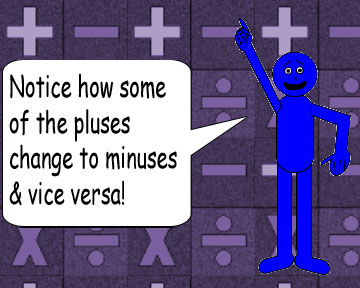# Complex Number Kookiness 5

## Observe the table below:

 Complex Number A Complex Number A-1 Complex Number B Complex Number B-1 1 + i 1/2 - 1/2i 1 - i 1/2 + 1/2i 2 + 2i 1/4 - 1/4i 2 - 2i 1/4 + 1/4i 3 + 3i 1/6 - 1/6i 3 - 3i 1/6 + 1/6i 4 + 4i 1/8 - 1/8i 4 - 4i 1/8 + 1/8i 5 + 5i 1/10 - 1/10i 5 - 5i 1/10 + 1/10i 6 + 6i 1/12 - 1/12i 6 - 6i 1/12 + 1/12i 7 + 7i 1/14 - 1/14i 7 - 7i 1/14 + 1/14i## This trick will still work even if X is a non-integer!

(½ + ½i)-1 = 1 - i

(½ - ½i)-1 = 1 + i

(1/3 + 1/3i)-1 = 1½ - 1½i

(1/3 - 1/3i)-1 = 1½ + 1½i

(√(2) + √(2)i)-1 = 1/(2√(2)) - 1/(2√(2))i

(√(2) - √(2)i)-1 = 1/(2√(2)) + 1/(2√(2))i

(π + πi)-1 = 1/(2π) - 1/(2π)i

(π - πi)-1 = 1/(2π) + 1/(2π)i## Just a warning: the variable X must be a positive number; otherwise, the plus or minus sign to the right of the equal sign WON'T change & you might add another minus sign to the real part of the dependent complex number, not to mention that addition & subtraction work differently when you involve negative numbers! Also, X can't be equal to zero(0) or you'll get a "division by zero" error on your calculator! Zero is the only number that has no other number as a reciprocal; it's infinity(∞), which ISN'T a number at all!